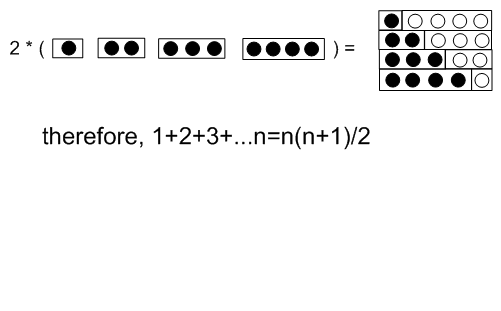# QED

QED: Beauty in Mathematical Proof by Burkard Polster is a short book with some elegant proofs (about 1 per page) from elementary geometry and number theory. Here are two that caught my eye. I know that .999…. =1 but I have always thought of this as something like a convention. Not so. Here is the proof.

Let x=.999…
then 10x=9.999…
now subtract x from both sides and we get
9x=9 or x=1

QED

And here is a just too lovely proof for the sum of the first n natural numbers.## Comments Sort by Top Sort by Recent Sort by Controversial

Comments for this post are closed